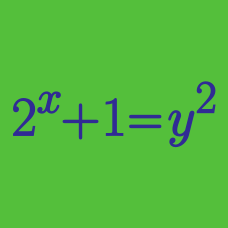Algebra

# Algebraic Manipulation - Substitution

If $x^{\frac{1}{2}}+x^{-\frac{1}{2}}=10,$ what is the value of $x+x^{-1}$?

Consider the polynomial $f(x)=x^3+ax+b$, where $a$ and $b$ are constants. If $f(x+1004)$ leaves a remainder of $52$ upon division by $x+1005$, and $f(x+1005)$ leaves a remainder of $6$ upon division by $x+1004$, what is the value of $a+b$?

The polynomial $x^n (x^2+ax+b)$ leaves a remainder of $9 ^n (x-9)$ upon division by $(x-9)^2,$ where $a$ and $b$ are constants and $n\geq 2$ is an integer. What is the value of $b-a$?

If $a = b + 4$, what is the value of

$3 a - 3 b + 3 ?$

If the equality $(x^2-2x+6)^3=a_6 x^6+a_5 x^5+\cdots+a_1 x^1+a_0$ always holds regardless the value of $x$, what is $a_0+a_2+a_4+a_6?$

×